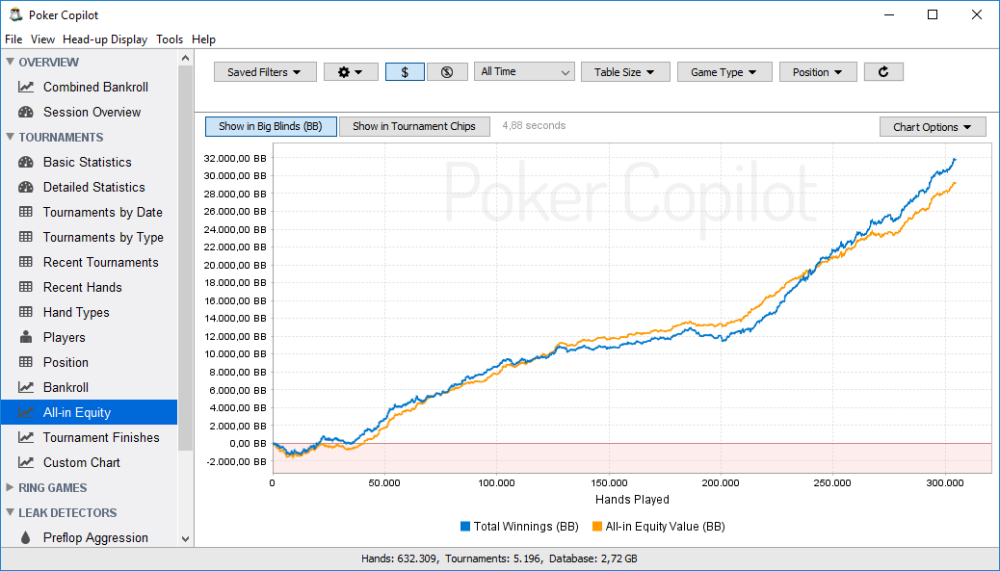To use the all-in equity chart you first need to understand the concept of “equity value.”

### What is “Equity Value”?

To help explain what equity value is, imagine the following scenario:
You are playing a hand of No Limit Texas Hold’em. You have AhQh and your opponent has KsKc. Your opponent raises to \$10 and is all-in. You call the bet, making the pot \$20.

Assume for this example that you and your opponent posted the blinds, all other players folded, and that there is no rake.

According to a product such as Poker Stove, the probability you will win this is hand is 32.1%. If you replayed this hand thousands of times, over the long run you would win 32.1% of the time. Your equity value is how much you would be likely to win per hand if you replayed this hand thousands of times, each time from a random deck. Therefore your equity value is 32.1% * \$20 = \$6.42

The formula for equity value (also known as pot equity) is:

Equity value = the probability you’ll win * (the total pot after the rake is removed)

### What is “Equity Value Difference”?

If you win the hand, you’ll win \$20. You’ll have exceeded your equity in the pot by \$20 – \$6.42 = \$13.58. Your Equity Value Difference is -\$13.58. If you lose the hand, you’ll be \$6.42 below your equity in the pot. Your Equity Value Difference is \$6.42.
The formula for Equity Value Difference is:

“Equity Value Difference” = your “equity value” – what you won = (the probability you’ll win * (total pot – rake)) – what you won

Remember this: when you lose an all-in hand, you’ll have a positive equity value difference. When you win an all-in hand and exceed pot equity, you’ll have a negative equity value difference. The exceptions are when you had 0% equity or 100% equity, in which case your equity value difference will be zero.

### Why is Equity Value only shown for all-in situations?

To properly calculate your “equity value” and “equity value difference” for a hand, we need to know your cards and your opponent’s cards. We can only be sure that this information is available in showdown hands. An all-in hand that gets called always goes to showdown, and so we can be sure we have enough information to calculate equity value. In other hands, we usually can’t determine enough information to make accurate calculations. Hence we talk of “All-in Equity” as a short way of saying “Equity Value” on hands in which you called an all-in bet, or in which your all-in bet was called.

### How do I read the All-in Equity chart?

In the short run, the All-in Equity chart is a crude measure of luck. Did you have some bad luck in the current session? Then your “Total Winnings” will be below your “All-in Equity Value” line. Did you get some lucky breaks? Then the “Total Winnings” line will be above the “All-in Equity Value” line.

In the long run (that is, over thousands of hands), the luck factor in the All-in Equity chart ceases to be relevant. Your “Total Winnings” line will converge to your “All-in Equity Value” line. If your expected EV graph is sloping up from left to right, you are making good reads. If your expected EV graph is sloping down from left to right, you are making bad reads.

You can change whether the All-In Equity chart shows in currency or big blinds by clicking the Show in \$ button and Show in BB button.Need more help with this?